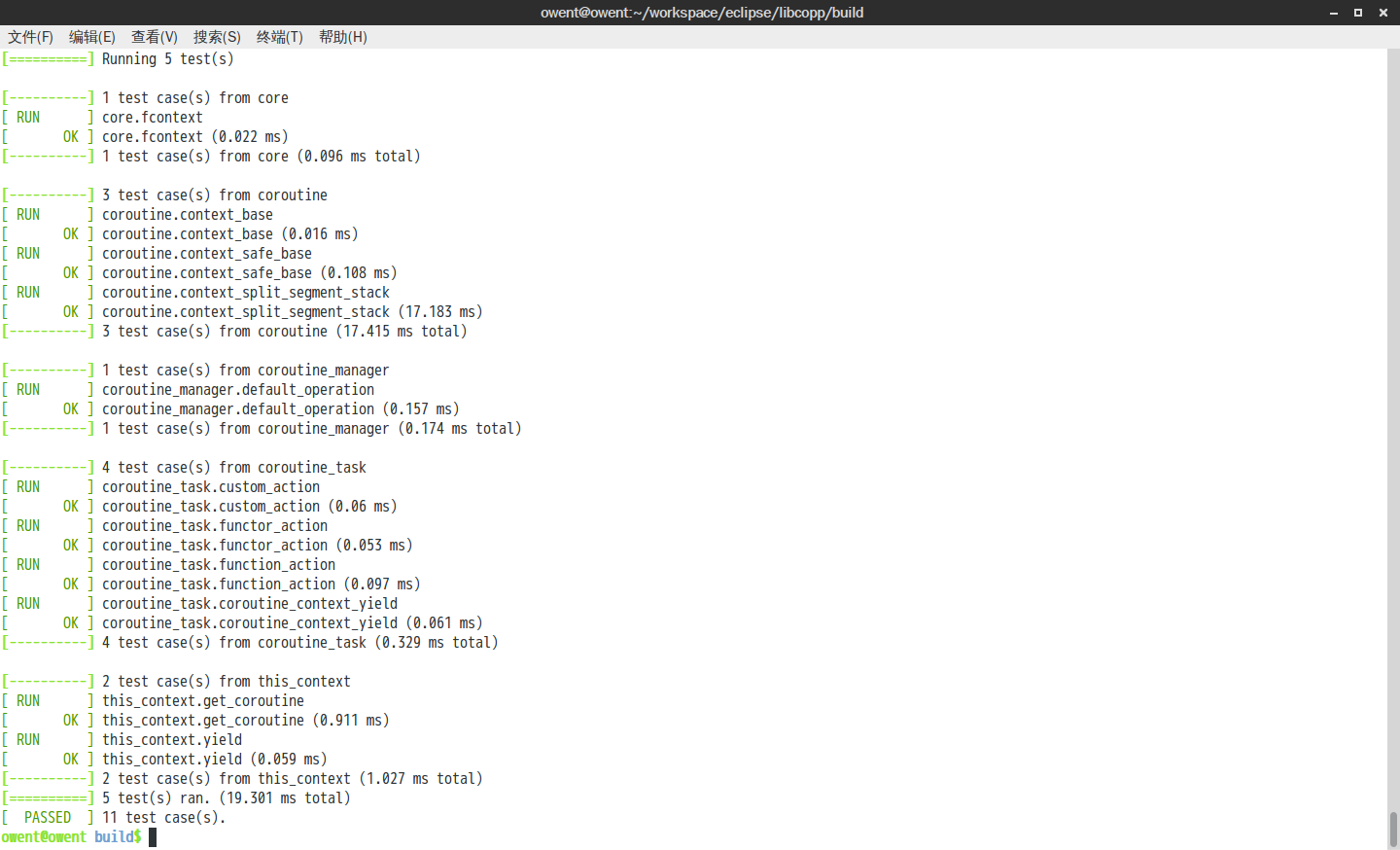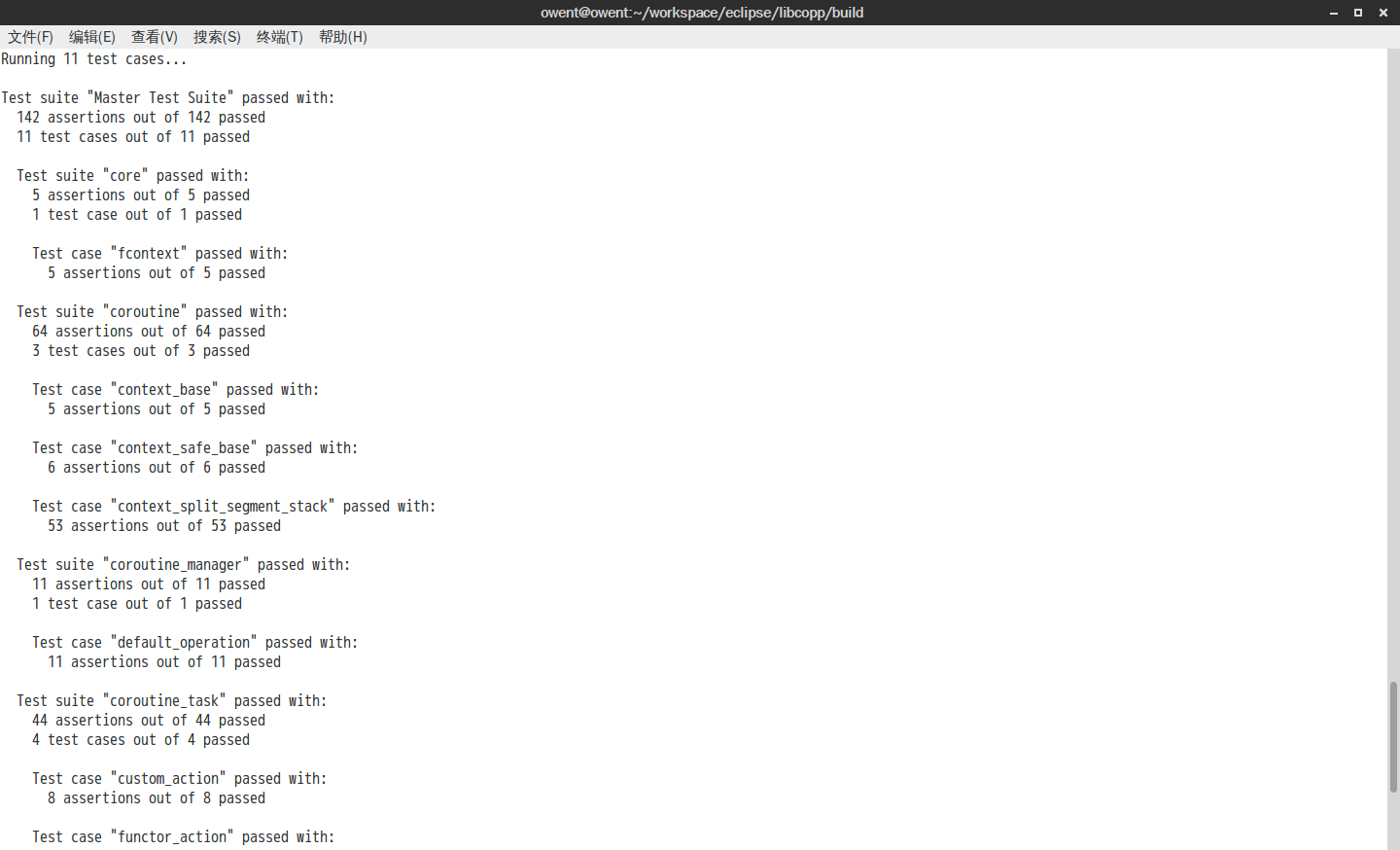# 简单C++单元测试框架(支持一键切到GTest或Boost.Test)

## 设计及技术方案

1. 在申明CASE_TEST的时候，先作函数声明

static void function_name();

1. 然后后跟一个全局注册变量，把suite名称、case名称和函数指针传进来

test_case_base g_var(suite名称, case名称, &function_name);

1. 最后跟上函数定义

void function_name()

## 一键切换适配方案 – GTest

#define test_case_func_name(test_name, case_name) test_func_test_##test_name##_case_##case_name
#define test_case_obj_name(test_name, case_name) test_obj_test_##test_name##_case_##case_name##_obj

#define CASE_TEST(test_name, case_name) \
static void test_case_func_name(test_name, case_name) (); \
static test_case_base test_case_obj_name(test_name, case_name) (#test_name, #case_name, test_case_func_name(test_name, case_name)); \
void test_case_func_name(test_name, case_name) ()

#define CASE_EXPECT_EXPR(expr) \
if(expr){ \
++(*test_manager::me().success_counter_ptr); \
} else { \
++(*test_manager::me().failed_counter_ptr);\
printf(shell_font::GenerateString("FAILED => %s:%d\nExpected: %s\n", SHELL_FONT_COLOR_RED).c_str(),\
__FILE__, __LINE__, \
#expr); \
}

#define CASE_EXPECT_TRUE(c) CASE_EXPECT_EXPR(c)
#define CASE_EXPECT_FALSE(c) CASE_EXPECT_EXPR(!(c))
#define CASE_EXPECT_EQ(l, r) CASE_EXPECT_EXPR((l) == (r))
#define CASE_EXPECT_NE(l, r) CASE_EXPECT_EXPR((l) != (r))
#define CASE_EXPECT_LT(l, r) CASE_EXPECT_EXPR((l) < (r))
#define CASE_EXPECT_LE(l, r) CASE_EXPECT_EXPR((l) <= (r))
#define CASE_EXPECT_GT(l, r) CASE_EXPECT_EXPR((l) > (r))
#define CASE_EXPECT_GE(l, r) CASE_EXPECT_EXPR((l) >= (r))


#define CASE_TEST(test_name, case_name) TEST(test_name, case_name)

#define CASE_EXPECT_TRUE(c) EXPECT_TRUE(c)
#define CASE_EXPECT_FALSE(c) EXPECT_FALSE(c)
#define CASE_EXPECT_EQ(l, r) EXPECT_EQ(l, r)
#define CASE_EXPECT_NE(l, r) EXPECT_NE(l, r)
#define CASE_EXPECT_LT(l, r) EXPECT_LT(l, r)
#define CASE_EXPECT_LE(l, r) EXPECT_LE(l, r)
#define CASE_EXPECT_GT(l, r) EXPECT_GT(l, r)
#define CASE_EXPECT_GE(l, r) EXPECT_GE(l, r)


## 一键切换适配方案 – Boost.Test

boost这个比较麻烦，因为boost的接口方式不一样，甚至在使用静态库的时候有自己的入口函数 简单地说就是分支比较多

1. 在入口处要判断是静态库还是动态库，有没有使用boost.test内置的函数（详见: https://github.com/owent/libcopp/tree/master/test/app/main.cpp
2. 添加suite和case的方式有变化（详见: https://github.com/owent/libcopp/tree/master/test/frame/test_manager.cpp
3. 还是宏定义的部分变更（详见: https://github.com/owent/libcopp/tree/master/test/frame/test_macros.h

## 效果展示

### 原版效果

cmake ../repo -DLIBCOPP_ENABLE_SEGMENTED_STACKS=YES
make -j4
test/coroutine_test### 一键切换到GTest效果

cmake ../repo -DLIBCOPP_ENABLE_SEGMENTED_STACKS=YES  -DGTEST_ROOT=/home/owent/workspace/libs/gtest
make -j4
test/coroutine_test### 一键切换到Boost.Test效果

cmake ../repo -DLIBCOPP_ENABLE_SEGMENTED_STACKS=YES -DLIBCOPP_TEST_ENABLE_BOOST_UNIT_TEST=YES -DBOOST_ROOT=/home/owent/workspace/libs/libboost
make -j4
test/coroutine_test -r detailed